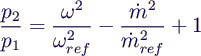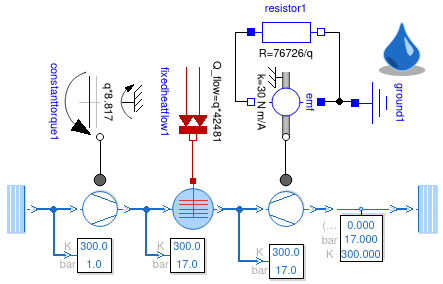### Joule-Brayton Process with Ideal Gas

• First Version:
• replace thermodynamic components
• define initial pressure and temperature
• mass flow not defined explicitly
• dynamical variable in ThermoDLR
• mainly depending on compressor parameters
• run → "Failed ... during initialization"
• Finding parameters for compressor and turbine:
• create simple test model Source - Compressor - Sink
• use input state, output pressure and torque from above  → doesn't run
• reason: completely different turbine characteristic
•• use formula to compute ω from mass flow and pressures
• use energy balance to compute τ
• use ω as initial value, and 0 for initial mass flow ("powering up")
• run → mass flow rises almost to correct value
• difference due to regularization in equations
• similar procedure for turbine
• quadratic equation has no real root!
• simple workaround: adapt parameter m_flow_ref
• Finishing steps:
• use computed parameters in initial version
• → runs and almost reproduces desired values
• finetuning
• change τ to get exact final pressure
• use scaling of all extensive parameters to get correct mass flow
• close the circle